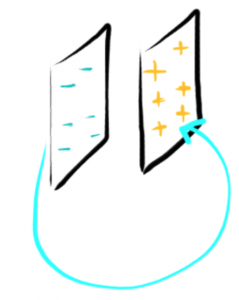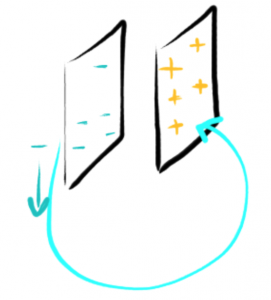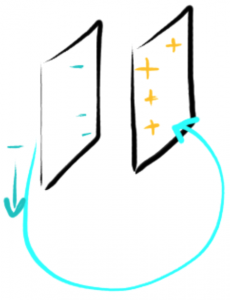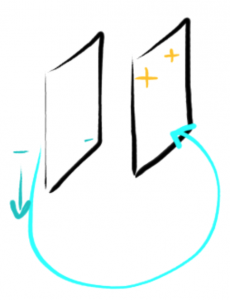# Capacitance

• Capacitors are used to store charge.
• The capacitance of a capacitor is the value that tells you how good a capacitor is at storing charge

Capacitance = Charge stored on a capacitor / Voltage across the capacitor

• Capacitance (C) = Q / V
• Q = Coulombs (c)
• V = Volt
• Coulomb / Volt = Farad (F)

Capacitors store Electrical Potential Energy:

• PEelectric = QV

But, Eof a capacitor = 1/2 QV

• Not all of the charges drop through the voltage V
• As charge decreases, the voltage across the capacitor decreases
• Only the first charge drops through voltage V

Here we have two plates with charge of -6, and +6; denoted with the plus and minus symbols.• The electrons are going to move to the positive plate
• Now, on the first go, the positive plate has 6/6 = 100%• On the third one, the positive plate only has 4/6 at the ‘time of departure’, which is 66%• The fifth one has 2/6 = 33%, and so on:• Thus, E = 1/2 QV